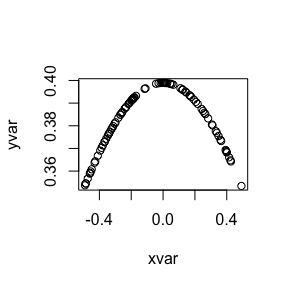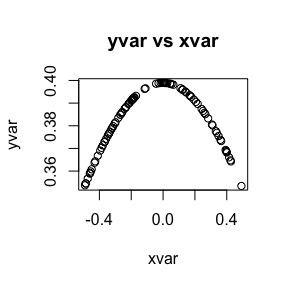# Let

#### 2020-12-06

The vignette demonstrates the use of let to standardize calls to functions that use non-standard evaluation. For a more formal description please see here.

For the purposes of this discussion, standard evaluation of variables preserves referential transparency: that is, values and references to values behave the same.

x = 5
print(5 + 1)
##  6
print(x + 1)
##  6

Some functions in R use non-standard evaluation (NSE) of variables, in order to snoop variable names (for example, plot), or to delay or even avoid argument evaluation (for example library(foobar) versus library("foobar")).

In the case of plot, NSE lets plot use the variable names as the axis labels.

set.seed(1234)
xvar = runif(100) - 0.5
yvar = dnorm(xvar)

plot(xvar, yvar)In the case of library, non-standard evaluation saves typing a couple of quotes. The dollar sign notation for accessing data frame columns also uses non standard evaluation.

d <- data.frame(x=c(1,NA))
d\$x
##   1 NA

Issues arise when you want to use functions that use non-standard evaluation – for brevity, I’ll call these NSE expressions – but you don’t know the name of the variable, as might happen when you are calling these expression from within another function. Generally in these situations, you are taking the name of the desired variable from a string. But how do you pass it to the NSE expression?

For this discussion, we will demonstrate let to standardize calling plot with unknown variables. let takes two arguments:

• A list of assignments symname=varname, where symname is the name used in the NSE expression, and varname is the name (as a string) of the desired variable.
• The NSE expression. Enclose a block of multiple expressions in brackets.

Here’s the plot example again.

library("wrapr")

xvariable = "xvar"
yvariable = "yvar"
let(
c(XVARIABLE=xvariable, YVARIABLE=yvariable),
{ # since we have the names as strings, we can create a title
title = paste(yvariable, "vs", xvariable)
plot(XVARIABLE, YVARIABLE, main=title)
}
)In the above let() block we are using the alias-convention that we specify substitution target names (in this case XVARIABLE and YVARIABLE) as upper-case analogues of the substitution name values (in this case xvariable and yvariable). This convention is very legible and makes it easy to both use value interfaces (as we did in the title paste()) and name-capturing interfaces (plot() itself).

## Implementation details

Roughly wrapr::let(A, B) behaves like a syntactic sugar for eval(substitute(B, A)).

a <- 1
b <- 2
let(c(z=quote(a)), z+b)
##  3
eval(substitute(z+b, c(z=quote(a))))
##  3

However, wrapr::let() is actually implemented in terms of a de-parse and safe language token substitution.

wrapr::let() was inspired by gtools::strmacro() and base::bquote(), please see here for some notes on macro methods in R.